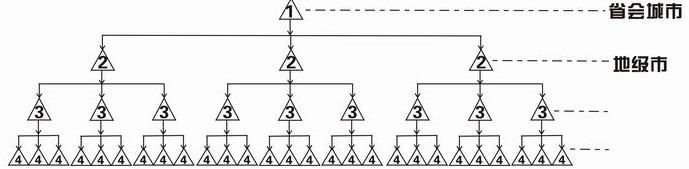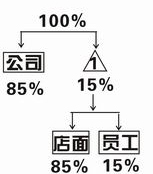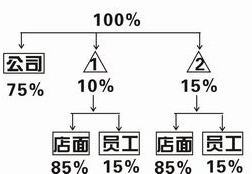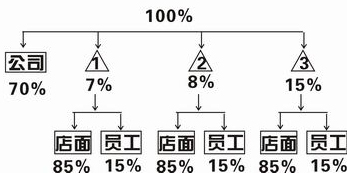1、 发展一个一级加盟店收取加盟费1万无；
2、 一级加盟店每发展一家二级加盟店，按加盟费0.85：0.15比例分配；加盟费：15000元 公司收入：15000*0.85=12750元 一级专卖店收入：15000*0.15=2250元 其中店面收入：2250*0.85=1912.5元 其中员工收入：2250*0.15=337.5元 (员工是指由小聪公司派出的技术员)

二级加盟店每发展一家三级加盟店，按加盟费0.75：0.10：0.15比例分配；加盟费：10000元 公司收入：10000*0.75=7500元 一级专卖店收入：10000*0.10=1000元 其中店面收入：1000*0.85=850元 其中员工收入：1000*0.15=150元 二级专卖店收入：10000*0.15=1500元 其中店面收入：1500*0.85=1275元 其中员工收入：1500*0.15=225元 (员工是指由小聪公司派出的技术员)

三级加盟店每发展一家四级加盟店，按加盟费0.70：0.07：0.08：0.15比例分配；加盟费：10000元 公司收入：10000*0.70=7000元 一级专卖店收入：10000*0.07=700元 其中店面收入：700*0.85=595元 其中员工收入：700*0.15=105元 二级专卖店收入：10000*0.08=800元 其中店面收入：800*0.85=680元 其中员工收入：800*0.15=120元 三级专卖店收入：10000*0.15=1500元 其中店面收入：1500*0.85=1275元 其中员工收入：1500*0.15=225元 (员工是指由小聪公司派出的技术员)

1、 小聪公司与一级加盟店按一级加盟店回款0.85：0.15比例分配；假设一级加盟店回款：10000元 公司收入：10000*0.85=8500元 一级专卖店收入：10000*0.15=1500元 其中店面收入：1500*0.85=1275元 其中员工收入：1500*0.15=225元 (员工是指由小聪公司派出的技术员)

2、 小聪公司、一级与二级加盟店按二级加盟店回款0.75：0.10：0.15比例分配；假设二级加盟店回款：10000元 公司收入：10000*0.75=7500元 一级专卖店收入：10000*0.10=1000元 其中店面收入：1000*0.85=850元 其中员工收入：1000*0.15=150元 二级专卖店收入：10000*0.15=1500元 其中店面收入：1500*0.85=1275元 其中员工收入：1500*0.15=225元 (员工是指由小聪公司派出的技术员)

3、小聪公司、一级、二级与三级加盟店按三级加盟店回款0.70：0.07：0.08：0.15比例分配；假设三级加盟店回款：10000元 公司收入：10000*0.70=7000元 一级专卖店收入：10000*0.07=700元 其中店面收入：700*0.85=595元 其中员工收入：700*0.15=105元 二级专卖店收入：10000*0.08=800元 其中店面收入：800*0.85=680元 其中员工收入：800*0.15=120元 三级专卖店收入：10000*0.15=1500元 其中店面收入：1500*0.85=1275元 其中员工收入：1500*0.15=225元 (员工是指由小聪公司派出的技术员)